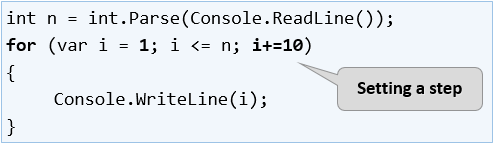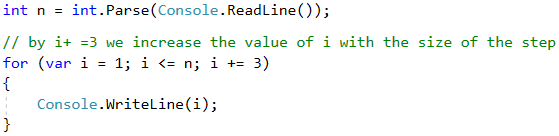# For Loop with Step

In the "Repetitions (Loops)" chapter we learned how the for loop works and we already know when and for what purpose to use it. In this chapter we will pay attention to a particular and very important part of its construction, namely the step.

## Video: Loop with a Step

Watch this video lesson to learn how to use for-loops with a custom step: https://youtu.be/QZDpWHcb7dE.

## Loop with a Step – Explanation

The step is that part of the for loop construction that tells how much to increase or decrease the value of its leading variable. It is declared the last in the skeleton of the for loop.

Most often, we have a size of 1, and in this case, instead of writing i += 1 or i -= 1, we can use the i++ or i-- operators. If we want our step to be different than 1, when increasing, we use the i += + step size operator, and when decreasing, the i -= + step size. With step of 10, the loop would look like this:Here is a series of sample problems, the solution of which will help us better understand the use of the step in for loop.

## Example: Numbers 1...N with Step 3

Write a program that prints the numbers from 1 to n with step of 3. For example, if n = 100, the result will be: 1, 4, 7, 10, …, 94, 97, 100.

We can solve the problem using the following sequence of actions (algorithm):

• We read the number n from the console input.
• We run a for loop from 1 to n with step size of 3.
• In the body of the loop, we print the value of the current step.